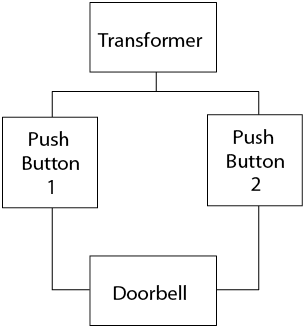# 2.2: Types of Electrical Diagrams

•• Contributed by Camosun College

There are four basic types of electrical diagrams:

• schematic
• wiring
• block
• pictorial

## Schematic Diagrams

The schematic diagram (Figure $$\PageIndex{1}$$), often called a ladder diagram, is intended to be the simplest form of an electrical circuit. This diagram shows the circuit components on horizontal lines without regard to their physical location. It is used for troubleshooting because it is easy to understand the operation of the circuit. The loads are located on the far right of the diagram and the controls for each load are located to the left. To understand the sequence of operation the drawing is read from the upper left corner and then from left to right and from top to bottom.Figure $$\PageIndex{1}$$: (CC BY-NC-SA; BC Industry Training Authority)
1. Schematic of a doorbell systemFigure $$\PageIndex{1}$$: (CC BY-NC-SA; BC Industry Training Authority)
2. Wiring diagramFigure $$\PageIndex{1}$$: (CC BY-NC-SA; BC Industry Training Authority)
3. Block diagramFigure $$\PageIndex{1}$$: (CC BY-NC-SA; BC Industry Training Authority)
4. Pictorial diagramNow complete the Learning Task Self-Test.